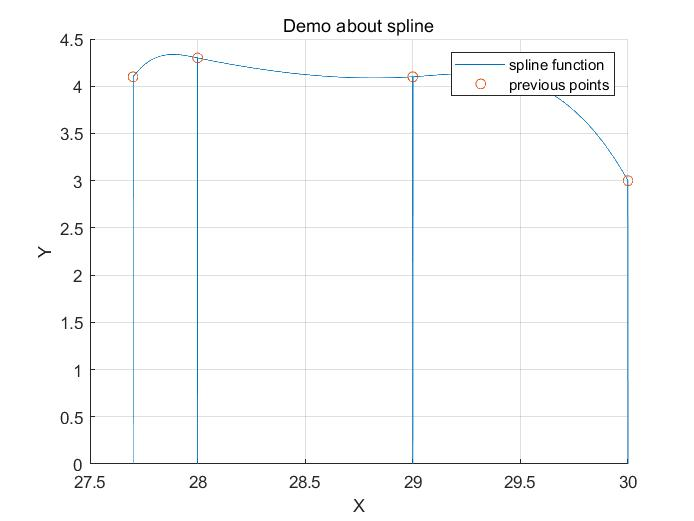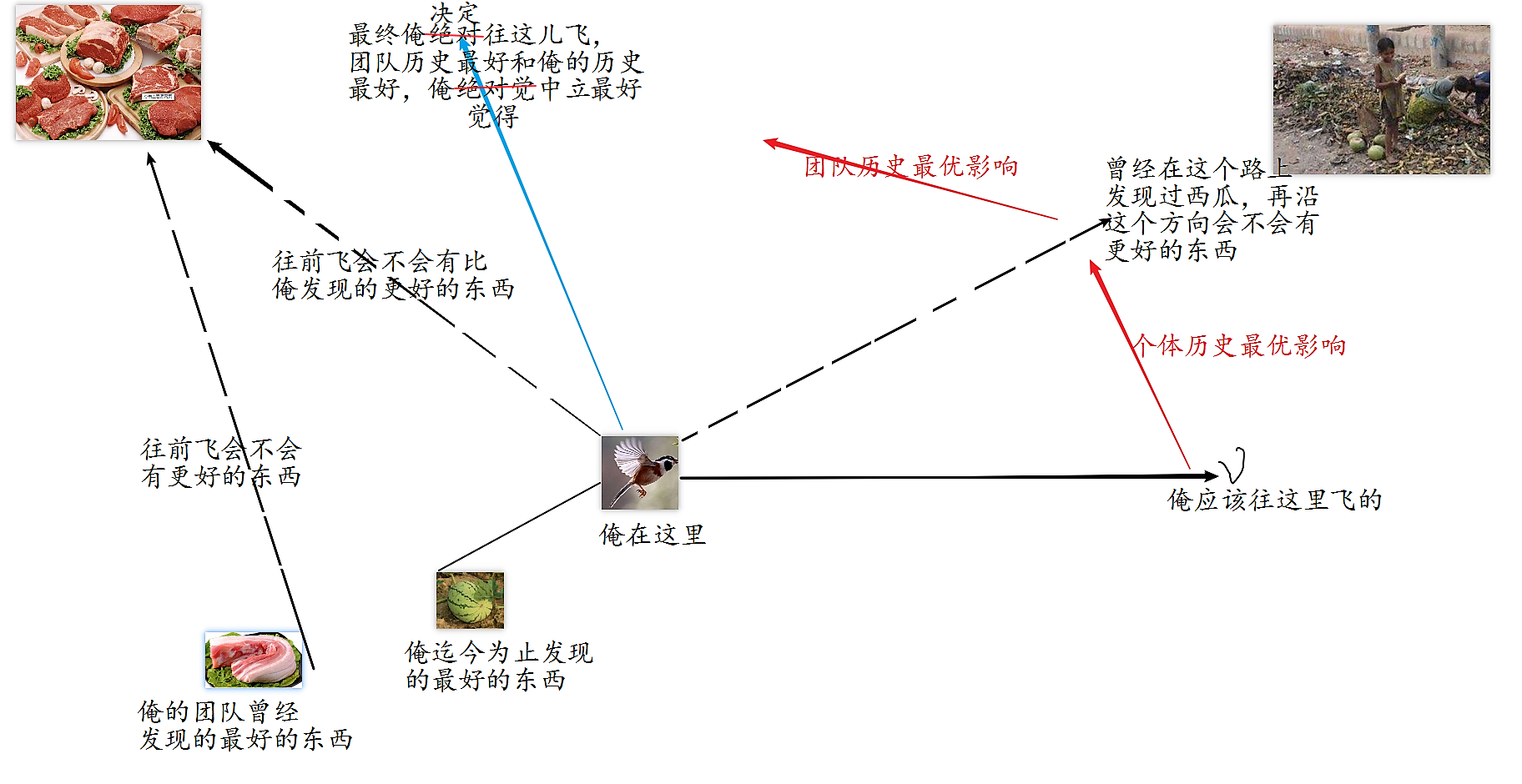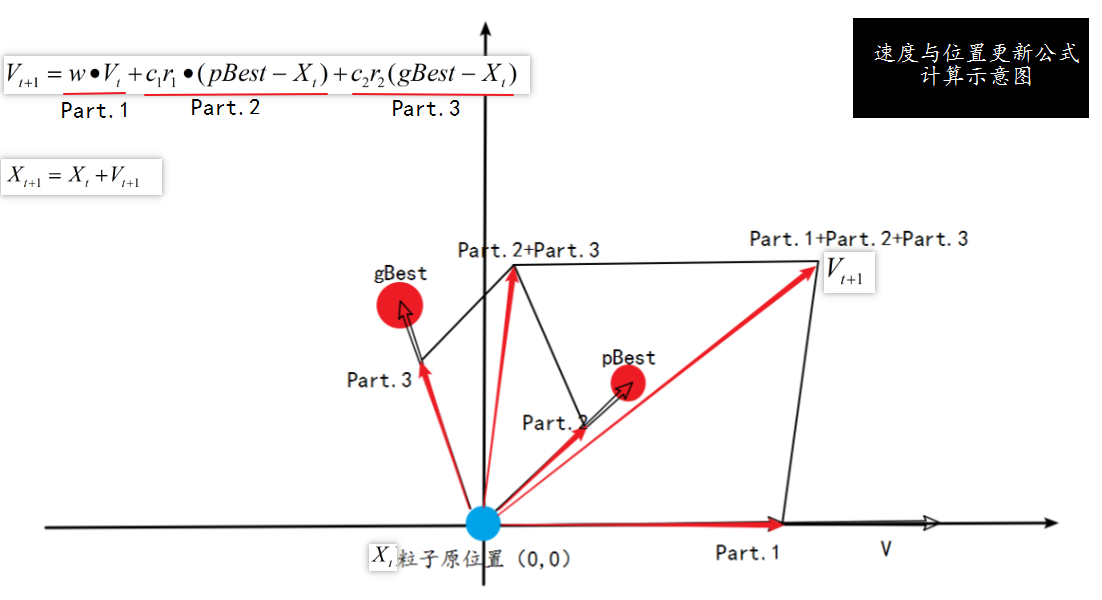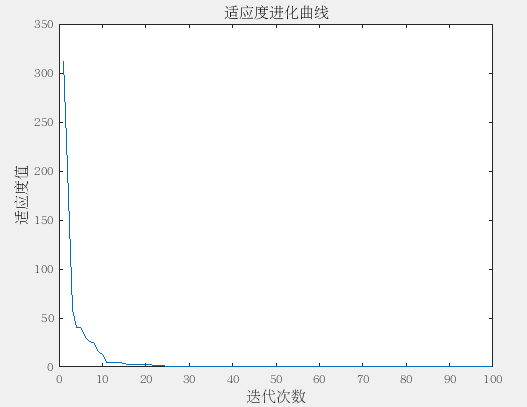• 来源：李庆杨等第五版《数值分析》P44页例题。目的：回顾一下matlab的基本用法。 首先根据书中给出的数学表达式编写... %Function：求解第一种三次样条函数插值 %变量说明： %Input varibles: % x：自变量数...

来源：李庆杨等第五版《数值分析》P44页例题。目的：回顾一下matlab的基本用法。

首先根据书中给出的数学表达式编写求解弯矩（书上这么叫的）函数：
function [M,A,d,H] = SolveSpline_1(x,y,diff_y0,diff_yn)
%Function：求解第一种三次样条函数插值
%变量说明：
%Input varibles:
% x：自变量数据（以列的形式输入）
% y：因变量数据（以列的形式输入）
% diff_y0：左端点导数值
% diff_yn：右端点到数值
%Output varibles:
%M：三次样条函数中的弯矩
%A：三对角矩阵
%d：右端向量
%H：节点步长

xx = x(2:end);
a = x(1);%作为x0点
[n,m] = size(xx);

A = 2*eye(n+1,n+1);%初始化三对角矩阵

H = diff(x);
%对角参数设置
mu = zeros(n,1);
mu(n) = 1;
for k = [1:n-1]
mu(k) = H(k)/(H(k) + H(k+1));
end

lambda = zeros(n,1);
lambda(1) = 1;
for k = [2:n]
lambda(k) = H(k)/(H(k-1) + H(k));
end

d = zeros(n+1,1);
%temp = (y(2) - y(1))/(x(2) - x(1))
d(1) = (6/H(1))*((y(2) - y(1))/(x(2) - x(1)) - diff_y0);
%temp = diff_yn - ((y(end) - y(end-1))/(x(end) - x(end-1)))
d(n+1) = (6/H(n))*(diff_yn - ((y(end) - y(end-1))/(x(end) - x(end-1))));
for k = [2:n]
%temp = (y(k+1) - y(k))/(x(k+1) - x(k))
%temp = (y(k) - y(k-1))/(x(k) - x(k-1))
d(k) = 6*((y(k+1) - y(k))/(x(k+1) - x(k)) - (y(k) - y(k-1))/(x(k) - x(k-1)))/(x(k+1) - x(k-1));
end

%给出三对角矩阵
A = A + diag(mu,-1) + diag(lambda,1);
%求解M
M = A^(-1)*d;
end

%这样求解完 M 后，便可将 M 带入到三次样条函数表达式中去，从而便得出了小区间上的三次样条插值函数

再编写主函数并绘制三次样条插值函数的图像，如下：
clc,clear all
x = [27.7,28,29,30]';
y = [4.1,4.3,4.1,3.0]';
diff_y0  = 3.0;
diff_yn = -4.0;
[M,A,d,H] = SolveSpline_1(x,y,diff_y0,diff_yn);

%利用分段函数的写法给出三次样条插值函数
[n,m] = size(x);
n = n-1;
t = [27.7:0.001:30];
S = 0;
for k = [1:n]

%{
temp = (x(k+1) - t).^3./(6*H(k))
temp = (t - x(k)).^3./(6*H(k))
temp = y(k) - M(k)*H(k)^2/6
temp = (x(k+1) - t)/H(k)
temp = y(k+1) - M(k+1)*H(k)^2/6
temp = x(k+1) - t
temp = (t - x(k+1))./H(k)
%}

S = S + (M(k)*((x(k+1) - t).^3./(6*H(k))) + M(k+1)*((t - x(k)).^3./(6*H(k))) ...
+ (y(k) - M(k)*H(k)^2/6).*((x(k+1) - t)/H(k)) + (y(k+1) - M(k+1)*H(k)^2/6).*((t - x(k))./H(k))).*(t > x(k) & t < x(k+1));
end

figure
hold on
plot(t,S);
scatter(x,y)
hold off
xlabel('X');
ylabel('Y');
legend('spline function','previous points')
grid on;

最后，简单的绘图结果如下：展开全文Matlab 数值分析
• 三次函数插值，若插值点为零怎么办？我们可以将其导函数取二次函数，通过矩阵方程，确定二次函数的系数和最值点，最后求积分可得所要的三次函数曲线
• Python 方程的种方法 （感谢前辈）转自：https://zhuanlan.zhihu.com/p/24893371 手抄一遍，加深印象。 评价：快速入门篇 Numpy 求解线性方程组 例如我们要解一个这样的二元一方程组： x + 2y = 3 4x ＋ 5y =...
Python 解方程的三种方法
（感谢前辈）转自：https://zhuanlan.zhihu.com/p/24893371
手抄一遍，加深印象。
评价：快速入门篇

Numpy 求解线性方程组
例如我们要解一个这样的二元一次方程组：
x + 2y = 3
4x ＋ 5y = 6

当然我们可以手动写出解析解，然后写一个函数来求解，这实际上只是用 Python 来单纯做“数值计算”. 但实际上，numpy.linalg.solve 可以直接求解线性方程组.
一般地，我们设解线性方程组形如 Ax=b，其中 A 是系数矩阵，b 是一维(n 维也可以，这个下面会提到)，x 是未知变量. 再拿上面地最简单的二元一次方程组为例，我们用 numpy.linalg.solve 可以这样写：
In : import numpy as np
...: A = np.mat('1,2; 4,5')    # 构造系数矩阵 A
...: b = np.mat('3,6').T       # 构造转置矩阵 b （这里必须为列向量）
...: r = np.linalg.solve(A,b)  # 调用 solve 函数求解
...: print r
...:
Out: [[-1.]
[ 2.]]

那么前面提到的“ n 维”情形是什么呢？实际上就是同时求解多组形式相同的二元一次方程组，例如我们想同时求解这样两组：
x + 2y = 3
4x ＋ 5y = 6
和

x + 2y = 7
4x ＋ 5y = 8

就可以这样写：
In : import numpy as np
...: A = np.mat('1,2; 4,5')          # 构造系数矩阵 A
...: b = np.array([[3,6], [7,8]]).T  # 构造转置矩阵 b （这里必须为列向量），
...: 注意这里用的是 array
...: r = np.linalg.solve(A,b)        # 调用 solve 函数求解
...: print r
...:
Out: [[-1.         -6.33333333]
[ 2.          6.66666667]]

SciPy 求解非线性方程组
先看官方文档的介绍：
scipy.optimize.fsolve(func, x0, args=(), fprime=None, full_output=0, col_deriv=0, xtol=1.49012e-08, maxfev=0, band=None, epsfcn=None, factor=100, diag=None)[source]

一般来说，我们只需要用到 func 和 x0 就够了. func 是自己构造的函数，也就是需要求解的方程组的左端（右端为 0），而 x0 则是给定的初值.
我们来看一个具体的例子，求解：
x + 2y + 3z - 6 = 0
5 * (x ** 2) + 6 * (y ** 2) + 7 * (z ** 2) - 18 = 0
9 * (x ** 3) + 10 * (y ** 3) + 11 * (z ** 3) - 30 = 0

就可以这么写：
In : from scipy.optimize import fsolve
...:
...: def func(i):
...:     x, y, z = i, i, i
...:     return [
...:             x + 2 * y + 3 * z - 6,
...:             5 * (x ** 2) + 6 * (y ** 2) + 7 * (z ** 2) - 18,
...:             9 * (x ** 3) + 10 * (y ** 3) + 11 * (z ** 3) - 30
...:            ]
...:
...: r = fsolve(func,[0, 0, 0])
...: print r
...:
Out: [ 1.00000001  0.99999998  1.00000001]

当然，SciPy 也可以用来求解线性方程组，这是因为 scipy.optimize.fsolve 本质上是最小二乘法来逼近真实结果.
SymPy 通吃一切
例如求解一个：
x + 2 * (x ** 2) + 3 * (x ** 3) - 6 = 0

直接就是：
In : from sympy import *
...: x = symbols('x')
...: solve(x + 2 * (x ** 2) + 3 * (x ** 3) - 6, x)
Out: [1, -5/6 - sqrt(47)*I/6, -5/6 + sqrt(47)*I/6]

另外， Wayne Shi 的这篇 使用 Python 解数学方程 ，就重点讲述了 SymPy 解线性方程组的方法，所以我也就不再赘述了。
其实 SymPy 能干的太多了，有兴趣的可以看一看 GitHub上的 Quick examples.
展开全文• 数值分析实验——三次样条插值 GoatWu 一、程序摘要 此程序使用 python3.7 语言编写。引入了外部库函数 numpy 作为数学工具方程，matplotlib 作为画图工具。由于需要多步运行，对不同的参数进行绘图，因此使用了 ...
数值分析实验——三次样条插值
GoatWu
一、程序摘要
此程序使用 python3.7 语言编写。引入了外部库函数 numpy 作为数学工具解方程，matplotlib 作为画图工具。由于需要多步运行，对不同的参数进行绘图，因此使用了 jupyter-notebook 作为编写工具。
由于用到的函数较多，为了安全起见，此程序将内部函数封装在了 Functions.py 模块中，将接口函数封装在了 spline.py 模块中。在 spline.py 中，我们引用了 Functions.py 的内部函数；在主程序中，我们只需要引入 spline 模块即可：import spline as sp。
程序有两个接口。第一个接口函数的功能是，对于给定的函数，在给定的范围中均匀选点，然后进行三次样条插值进行拟合；第二个接口函数的功能是，对于给定的点集与边界条件，将这些点利用三次样条插值进行拟合。下面我们将逐一呈现。
二、程序功能
1. 给定函数的拟合
接口：sp.give_func(func = F.runge, beg = -5, end = 5, segments = 10)
参数意义：
func：需要拟合的函数。默认值为 Functions 中的龙格函数beg：拟合范围的左端点end：拟合范围的右端点segments：拟合的区间个数，也即点的个数减

1

1

使用示例：
sp.give_func()：
在

[

−

5

,

5

]

[-5,5]

的范围内用

10

10

段拟合龙格函数sp.give_func(beg = -5, end = 5, segments = 6)：
在

[

−

5

,

5

]

[-5,5]

的范围内用

6

6

段拟合龙格函数。def f(x):
return (x * x * x + 6 * x * x + 7 * x + 1) / (x * x + 1);
sp.give_func(f, -4, 4, 8)

对自定义的函数

f

(

x

)

=

x

3

+

6

x

2

+

7

x

+

1

x

2

+

1

f(x)=\frac{x^3+6x^2+7x+1}{x^2+1}

，分

8

8

段 在区间

[

−

4

,

4

]

[-4,4]

来拟合函数。2. 给定点集的拟合
接口：sp.give_nodes(x, y, opt = 0, lval = 0, rval = 0)
参数意义：
x：给定点集的

x

x

坐标数组y：给定点集的

y

y

坐标数组opt：插值的边界条件
opt = 0：默认边界条件，左右两端点处的三阶导与前一端点处三阶导相等opt = 1：给定左右两端点的一阶导，分别为lval 和rval 。opt = 2：给定左右两端点的二阶导，分别为lval 和rval 。
使用示例：
x = x = [27.7, 28, 29, 30]
y = [4.1, 4.3, 4.1, 3.0]

sp.give_nodes(x, y)：
对给定的点集用默认边界条件来拟合。sp.give_nodes(x, y, opt = 1, lval = 3.0, rval = -4.0)：
给定左右边界端点的一阶导来拟合。sp.give_nodes(x, y, opt = 2, lval = 27.0, rval = -34.0)：
给定左右边界端点的二阶导来拟合。3. 给出各分段的三次函数表达式三、程序的鲁棒性
1. 无给定函数的提示
对于给定函数的拟合，在无输入函数的情况下，默认使用龙格函数，并弹出提示：2. 错误的 opt 参数
对于给定点集的拟合，如果 opt 参数不为有意义的值，返回错误：3. 额外的 lval 或 rval 值
对于给定点集的拟合，如果 opt = 0 下，仍给出lval 或 rval 值，返回错误：4. 给定点集的 x 和 y 长度不等
对于给定点集的拟合，如果 x 数组和 y 数组长度不等，返回错误：四、部分程序运行结果
1. 给定函数的拟合
1.1. 龙格函数1.2. 给定其他函数2. 给定点集的拟合
2.1. 默认情况2.2. 给定一阶导的边界条件2.3. 给定二阶导的边界条件五、程序源代码
1. Functions.py
import math
import numpy as np
import matplotlib.pyplot as plt

def runge(y):
y = np.float32(y)
return 1/(1 + y * y)

def spline3_Parameters(x_vec, opt = 0):
# 建立三对角矩阵的 4n 个方程的左边部分
# parameter为二维数组，用来存放参数，size_of_Interval为区间的个数
x_new = np.array(x_vec)
parameter = []
size_of_Interval = len(x_new) - 1;
i = 1
# 相邻两区间公共节点处函数值相等的方程，共2n-2个
while i < len(x_new) - 1:
data = np.zeros(size_of_Interval * 4)
data[(i - 1) * 4] = x_new[i] * x_new[i] * x_new[i]
data[(i - 1) * 4 + 1] = x_new[i] * x_new[i]
data[(i - 1) * 4 + 2] = x_new[i]
data[(i - 1) * 4 + 3] = 1
parameter.append(data)
data = np.zeros(size_of_Interval * 4)
data[i * 4] = x_new[i] * x_new[i] * x_new[i]
data[i * 4 + 1] = x_new[i] * x_new[i]
data[i * 4 + 2] = x_new[i]
data[i * 4 + 3] = 1
parameter.append(data)
i += 1
# 左右端点处的函数值。为两个方程, 加上前面的2n-2个方程，一共2n个方程
data = np.zeros(size_of_Interval * 4)
data = x_new * x_new * x_new
data = x_new * x_new
data = x_new
data = 1
parameter.append(data)
data = np.zeros(size_of_Interval * 4)
data[(size_of_Interval - 1) * 4] = x_new[-1] * x_new[-1] * x_new[-1]
data[(size_of_Interval - 1) * 4 + 1] = x_new[-1] * x_new[-1]
data[(size_of_Interval - 1) * 4 + 2] = x_new[-1]
data[(size_of_Interval - 1) * 4 + 3] = 1
parameter.append(data)
# 端点函数一阶导数值相等为n-1个方程。加上前面的方程为3n-1个方程。
i = 1
while i < size_of_Interval:
data = np.zeros(size_of_Interval * 4)
data[(i - 1) * 4] = 3 * x_new[i] * x_new[i]
data[(i - 1) * 4 + 1] = 2 * x_new[i]
data[(i - 1) * 4 + 2] = 1
data[i * 4] = -3 * x_new[i] * x_new[i]
data[i * 4 + 1] = -2 * x_new[i]
data[i * 4 + 2] = -1
parameter.append(data)
i += 1
# 端点函数二阶导数值相等为n-1个方程。加上前面的方程为4n-2个方程。
i = 1
while i < len(x_new) - 1:
data = np.zeros(size_of_Interval * 4)
data[(i - 1) * 4] = 6 * x_new[i]
data[(i - 1) * 4 + 1] = 2
data[i * 4] = -6 * x_new[i]
data[i * 4 + 1] = -2
parameter.append(data)
i += 1

# 两个附加条件
# 默认情况：opt = 0，not-a-knot边界条件：左边两端点三阶导相等，右边两端点三阶导也相等
if opt == 0:
data = np.zeros(size_of_Interval * 4)
data = 6
data = -6
parameter.append(data)
data = np.zeros(size_of_Interval * 4)
data[-4] = 6
data[-8] = -6
parameter.append(data)
# opt = 1，给定左右两端点的一阶导值
if opt == 1:
data = np.zeros(size_of_Interval * 4)
data = 3 * x_new * x_new
data = 2 * x_new
data = 1
parameter.append(data)
data = np.zeros(size_of_Interval * 4)
data[-4] = 3 * x_new[-1] * x_new[-1]
data[-3] = 2 * x_new[-1]
data[-2] = 1
parameter.append(data)
# opt = 2，给定左右两端点的二阶导值
if opt == 2:
data = np.zeros(size_of_Interval * 4)
data = 6 * x_new
data = 2
parameter.append(data)
data = np.zeros(size_of_Interval * 4)
data[-4] = 6 * x_new[-1]
data[-3] = 2
parameter.append(data)

return parameter

def solution_of_equation(functype, parametes, x, y = 0, func = runge, opt = 0, lval = 0, rval = 0):
# 建立三对角线性方程组并求解，得到各段三次函数的系数并返回
# functype 表示需要拟合的是给定函数 / 给定点集
size_of_Interval = len(x) - 1;
result = np.zeros(size_of_Interval * 4)
i = 1
if functype != 'give_func' and functype != 'give_nodes':
raise ValueError("functype should be 'give_func' or 'give_nodes' ")

if functype == 'give_func':
while i < size_of_Interval:
result[(i - 1) * 2] = func(x[i])
result[(i - 1) * 2 + 1] = func(x[i])
i += 1
result[(size_of_Interval - 1) * 2] = func(x)
result[(size_of_Interval - 1) * 2 + 1] = func(x[-1])

if functype == 'give_nodes':
if len(x) != len(y):
raise ValueError("Expect a node set!")
while i < size_of_Interval:
result[(i - 1) * 2] = y[i]
result[(i - 1) * 2 + 1] = y[i]
i += 1
result[(size_of_Interval - 1) * 2] = y
result[(size_of_Interval - 1) * 2 + 1] = y[-1]

# 默认情况：opt = 0，not-a-knot边界条件：左边两端点三阶导相等，右边两端点三阶导也相等
if opt == 0:
result[-2] = result[-1] = 0;
# opt = 1 或 opt = 2，给定左右两端点的一阶导值 / 二阶导值
if opt == 1 or opt == 2:
result[-2] = lval
result[-1] = rval

a = np.array(parametes)
b = np.array(result)
return np.linalg.solve(a, b)

def calculate(paremeters, x):
# 计算x在拟合得到的函数中的点值
res = []
for dx in x:
res.append(paremeters * dx * dx * dx + paremeters * dx * dx + paremeters * dx + paremeters)
return res

def draw_pic(functype, x, y, func = runge, xnd = 0, ynd = 0):
fig = plt.figure()
plt.plot(x, y, label='interpolation')
if functype == 'give_func':
plt.plot(x, func(x), label='raw')
l = len(xnd)
for i in range(0, l):
plt.plot(xnd[i], ynd[i], 'bo')
plt.legend()
plt.show()
plt.close(fig)

2. spline.py
import Functions as F
import numpy as np

def give_func(func = F.runge, beg = -5, end = 5, segments = 10):

if func == F.runge:
print("warning: No function input! So we use the default function Runge Function\n")

interval = 1.0 * (end - beg) / segments
x_init4 = np.arange(beg, end + 0.0001, interval)
res = F.solution_of_equation('give_func', F.spline3_Parameters(x_init4), x_init4, y = 0, func = func)
x_axis4 = []
y_axis4 = []
xnd = []
ynd = []
for i in range(segments):
temp = np.arange(beg + i * interval, beg + (i + 1) * interval, 0.01)
xid = beg + i * interval
xnd = np.append(xnd, xid)
ynd = np.append(ynd, F.calculate([res[4 * i], res[1 + 4 * i], res[2 + 4 * i], res[3 + 4 * i]], np.array([xid])))
x_axis4 = np.append(x_axis4, temp)
y_axis4 = np.append(y_axis4, F.calculate([res[4 * i], res[1 + 4 * i], res[2 + 4 * i], res[3 + 4 * i]], temp))

i = segments - 1
xid = beg + (i + 1) * interval
xnd = np.append(xnd, xid)
ynd = np.append(ynd, F.calculate([res[4 * i], res[1 + 4 * i], res[2 + 4 * i], res[3 + 4 * i]], np.array([xid])))

for i in range(len(xnd) - 1):
print(f"x in [{xnd[i]:.3f}, {xnd[i+1]:.3f}]: S(x) = {res[4*i]:.3f}x^3 + {res[1+4*i]:.3f}x^2 + {res[2+4*i]:.3f}x + {res[3+4*i]:.3f}")

F.draw_pic("give_func", x_axis4, y_axis4, func, xnd, ynd)

def give_nodes(x, y, opt = 0, lval = 0, rval = 0):

if opt == 0 and (lval != 0 or rval != 0):
raise ValueError('There should be no parameters of lval and rval by default')
if opt != 0 and opt != 1 and opt != 0 and opt != 2:
raise ValueError('opt should be 0 or 1 or 2!')

if opt == 0:
res = F.solution_of_equation('give_nodes', F.spline3_Parameters(x), x, y)
if opt == 1:
res = F.solution_of_equation('give_nodes', F.spline3_Parameters(x, 1), x, y, opt = 1, lval = lval, rval = rval)
if opt == 2:
res = F.solution_of_equation('give_nodes', F.spline3_Parameters(x, 2), x, y, opt = 2, lval = lval, rval = rval)

for i in range(len(x) - 1):
print(f"x in [{x[i]:.3f}, {x[i+1]:.3f}]: S(x) = {res[4*i]:.3f}x^3 + {res[1+4*i]:.3f}x^2 + {res[2+4*i]:.3f}x + {res[3+4*i]:.3f}")

x_axis4 = []
y_axis4 = []
for i in range(len(x) - 1):
temp = np.arange(x[i], x[i + 1], 0.01)
x_axis4 = np.append(x_axis4, temp)
y_axis4 = np.append(y_axis4, F.calculate([res[4 * i], res[1 + 4 * i], res[2 + 4 * i], res[3 + 4 * i]], temp))
F.draw_pic("give_nodes", x_axis4, y_axis4, func = None, xnd = x, ynd = y)


3. main.py
以下代码最好在 jupyter-notebook 中分条运行！如果是在 pycharm 等 ide 下或者是 sublime 等文本编辑器，需要将其余代码注释掉再分别运行。
import spline as sp

# 接口函数一：give_func(func = function, beg = -5, end = 5, segments = 10)
# 函数意义：对给定的函数，在 [beg, end] 范围内进行拟合，共 segments 段（即 segments+1 个点）
# 参数意义：func表示需要拟合的函数，默认为龙格函数；
#         beg、end表示插值的范围，segments表示插值的数量。默认值写在上面。

sp.give_func()
sp.give_func(beg = -5, end = 5, segments = 6)

def f(x):
return (x * x * x + 6 * x * x + 7 * x + 1) / (x * x + 1);
sp.give_func(f, -4, 4, 8)

# 接口函数二：give_nodes(x, y, opt = 0, lval = 0, rval = 0)
# 函数意义：对于给定的点集进行插值
# 参数意义：x,y 表示点集的坐标；
# opt表示边界条件的处理：
# opt = 0 表示默认边界条件，not-a-knot边界条件：左边两端点三阶导相等，右边两端点三阶导也相等
# opt = 1 或 opt = 2，表示给定左右两端点的一阶导值 / 二阶导值

x = x = [27.7, 28, 29, 30]
y = [4.1, 4.3, 4.1, 3.0]
sp.give_nodes(x, y)
sp.give_nodes(x, y, opt = 1, lval = 3.0, rval = -4.0)
sp.give_nodes(x, y, opt = 2, lval = 27.0, rval = -34.0)

六、总结
此程序仅仅调用了 python 的外部库函数 numpy 用于解三对角矩阵方程，matplotlib 用于绘图，具有较好的独立性；兼容常用的两种边界条件，并且仿照 Matlab 自带的三次样条插值，设置了默认的 “两边三阶导与前一节点三阶导相等” 的边界条件；做了良好的封装，内部函数封装在 Functions 中不可被调用，并且支持多种的插值方式，对于错误的输入也能给予响应，具有较好的通用性。此程序的复杂度瓶颈在于解三对角矩阵方程，由于 numpy 强大的性能，也拥有良好的运行效率，在感受上运行几乎无延迟。
展开全文python 算法
• 例 ： 求解函数最小值 粒子群算法的驱动因素 从鸟群觅食行为到粒子群算法 鸟群寻找食物的过程中，鸟与鸟之间存在着信息的交换，每只鸟搜索目前离食物最近的鸟的周围区域是找到食物的最简单有效的办法。 ...


文章目录
@[toc]
从鸟群觅食行为到粒子群算法粒子群算法的核心例 ： 求解函数最小值粒子群算法的驱动因素

从鸟群觅食行为到粒子群算法鸟群寻找食物的过程中，鸟与鸟之间存在着信息的交换，每只鸟搜索目前离食物最近的鸟的周围区域是找到食物的最简单有效的办法。
粒子群算法（以下简称PSO）就是模拟鸟群觅食行为的一种彷生算法 。 解=粒子=鸟 (鸟的位置象征着离食物的距离，粒子的位置也象征着离最优解的距离，是评价解质量的唯一标准)， 找食物=找最优解，一个西瓜=一个粒子找到的历史最优解，一块肉=整个粒子群找到历史最优解 ，

就像鸟的飞行路线会受到自己曾经寻找到的最优食物和鸟群曾经找到过的最优食物的双重影响一样，算法中，每一次迭代，粒子通过两个"极值"(全局历史最优解gBest和个体历史最优解pBest)来更新自己的速度，该速度又是更新粒子位置的关键，而粒子的位置象征着离最优解的距离，也是评价该粒子(解)的唯一标准 。

粒子群算法的核心
该算法的核心是如何根据pBest与gBest来更新粒子的速度和位置，标准粒子群给出了如下的更新公式：

$V_{t+1} =w \cdot V_t +c_1r_1\cdot(pBest-X_t) +c_2r_2\cdot(gBest-X_t)$

X

t

+

1

=

X

t

+

V

t

+

1

X_{t+1} = X_t+V_{t+1}

$其中 ， t：代数 ， X是位置，V是速度，w是惯性权重，c是学习因子，r是随机数$如上图所示，假设这是一个在2维平面内寻找最优解的待求解问题，某一时间的某一粒子

X

t

X_t

处在原点位置 。则该粒子更新后的速度如上图所示 。 更新公式可以分为三个部门：
Part.1 : "惯性"或"动量"部分，反映粒子有维持自己先前速度的趋势Part.2 : "认知"部门 ， 反映粒子有向自身历史最优位置逼近的趋势Part.3 : "社会"部门 ， 反映粒子有向去群体历史最优位置逼近的趋势
例 ： 求解函数最小值

​ 求$f(x)=\sum_{i=1}{n}x_i2,(-20 \leq x\leq 20，n=10)$ 的最小值 ?

% author zhaoyuqiang
clear all ;
close all ;
clc ;
N = 100 ; %　种群规模
D = 10 ; % 粒子维度
T = 100 ; %　迭代次数
Xmax = 20 ;
Xmin = -20 ;
C1 = 1.5 ; %　学习因子1
C2 = 1.5 ; %　学习因子2
W = 0.8 ; %　惯性权重
Vmax = 10 ; %　最大飞行速度
Vmin = -10 ; %　最小飞行速度
popx = rand(N,D)*(Xmax-Xmin)+Xmin ; % 初始化粒子群的位置(粒子位置是一个D维向量)
popv = rand(N,D)*(Vmax-Vmin)+Vmin ; % 初始化粒子群的速度(粒子速度是一个D维度向量)
% 初始化每个历史最优粒子
pBest = popx ;
pBestValue = func_fitness(pBest) ;
%初始化全局历史最优粒子
[gBestValue,index] = max(func_fitness(popx)) ;
gBest = popx(index,:) ;
for t=1:T
for i=1:N
% 更新个体的位置和速度
popv(i,:) = W*popv(i,:)+C1*rand*(pBest(i,:)-popx(i,:))+C2*rand*(gBest-popx(i,:)) ;
popx(i,:) = popx(i,:)+popv(i,:) ;
% 边界处理，超过定义域范围就取该范围极值
index = find(popv(i,:)>Vmax | popv(i,:)<Vmin);
popv(i,index) = rand*(Vmax-Vmin)+Vmin ; %#ok<*FNDSB>
index = find(popx(i,:)>Xmax | popx(i,:)<Xmin);
popx(i,index) = rand*(Xmax-Xmin)+Xmin ;
% 更新粒子历史最优
if func_fitness(popx(i,:))>pBestValue(i)
pBest(i,:) = popx(i,:) ;
pBestValue(i) = func_fitness(popx(i,:));
end
if pBestValue(i) > gBestValue
gBest = pBest(i,:) ;
gBestValue = pBestValue(i) ;
end
end
% 每代最优解对应的目标函数值
tBest(t) = func_objValue(gBest); %#ok<*SAGROW>
end
figure
plot(tBest);
xlabel('迭代次数') ;
ylabel('适应度值') ;
title('适应度进化曲线') ;粒子群算法的驱动因素
粒子群算法是一种随机搜索算法 。粒子的下一个位置受到自身历史经验和全局历史经验的双重影响，全局历史经验时刻左右着粒子的更新，群体中一旦出现新的全局最优，则后面的粒子立马应用这个新的全局最优来更新自己，大大提高了效率，相比与一般的算法(如遗传算法的交叉)，这个更新过程具有了潜在的指导，而并非盲目的随机 。
自身历史经验和全局历史经验的比例尤其重要，这能左右粒子的下一个位置的大体方向，所以，粒子群算法的改进也多种多样，尤其是针对参数和混合其他算法的改进 。

总体来说，粒子群算法是一种较大概率收敛于全局最优解的，适合在动态、多目标优化环境中寻优的一种高效率的群体智能算法。

展开全文粒子群算法 matlab源码 粒子群更新公式 智能计算
• 求一元二方程的，考虑所有情况 假设：ax²+bx+c=0 要求输入a,b,c的值，判断并求出方程的。 有以下几种情况： 1、a = 0 （1）b = 0 　c = 0时，x可以是任意数； 　c != 0时，方程不成立； （2）b != 0 　方程为...C语言
• MATLAB实现一元三次方程求解/盛金公式 一元三次方程求解中，1945年卡尔丹诺把冯塔纳的三次方程求根公式发表出来，但该公式形式比较复杂，直观性也较差。1989年范盛金对一元三次方程求解进行了深入的研究和探索，提出...盛金公式 MATLAB代码
• ## MATLAB函数速查手册

千次阅读 多人点赞 2018-03-25 09:06:26matlab
• 使用方法： roots(num) 其中，num是方程的系数数组，并且按照自变量幂降序排列 示例： 求解方程3x^2-2x-4=0的根 >> roots([3 -2 -4]) ans = 1.5352 -0.8685
• 请定义一个函数quadratic(a, b, c)，接收3个参数，返回一元二方程：ax² + bx + c = 0的两个。 过程 分析 此题主要考的是对一元二方程的理解，首先a不能为0，其次再分析b**2-4*a*c,若b**2-4*a*c&lt;0无实...Python
• Matlab solve函数计算三元一方程组实例：计算状态转移率 实例：计算状态转移率 %matlab代码 clc; clear; Lambda = 0.0001; Mu = 2; syms p0 p1 p2; %定义个状态中处于某个状态的概率 [solp0,solp1,solp2] = ...matlab
• 数值分析用matlab求解三次样条插值多项式 时间真快，2018年只剩下2天，2019年即将来临！ 今晚整理笔记本中的资料，看了下之前给朋友解答的一个《数值分析》实验题目，还是有点意思。不管怎样，分享给需要的朋友，...matlab 数值分析
• ## matlab解方程、方程组

万次阅读 多人点赞 2016-06-23 17:11:03
1、方程、方程组 x^2-4=12,求x： syms x; f=x^2-4-12; solve(f) 最近有多人问如何用matlab方程组的问题，其实在matlab中方程组还是很方便的，例如，对于代数方程组Ax=b(A为系数矩阵，非奇异)的...
• ## 三次样条插值Python实现

万次阅读 热门讨论 2018-06-04 22:15:20
在每个分段区间上是三次多项式（这就是三次样条中的三次的来源） 在整个区间（开区间）上二阶导数连续（当然啦，这里主要是强调在节点上的连续） 加上边界条件。边界条件只需要给出两个方程。构建一个方程组，就...数值分析 python
• 实现三次样条插值，给定从Xo到Xn的点，再给定边界条件，运用数学方法求出该三次样条函数。其中，边界条件有两种，第一种：给定边界的一阶导数；第二种：给定边界的二阶导数。 二：实验工具 MATLAB 三.实验思路 实验...
• #include #include int main() { double a,b,c,d,e,x1,x2; printf("请输入ax^2+bx+c=0中a b c的值"); scanf("%lf,%lf,%lf",&a,&b,&c); e=b*b-4*a*c;...printf("无，请重新输入\n");  scanf("%lfC语言 编程 c语言Python python
• import math def quadratic(a,b,c): L =[a,b,c] for i in L: if not isinstance(i,(float,int)): print('数据类型有误') delta=b*b-4*a*c if delta&lt;0: print('无') return e...Python
• 实现一个求解一元二方程的类，该类包含个成员变量和一个求解一元二方程函数，该函数需要抛出异常（1.无的异常 2二项系数为0的异常））抛出异常
• ## 三次样条插值介绍

千次阅读 2019-08-24 20:31:48
线性拟合会存在一个问题，拟合出来的函数不够“光滑”，为了让线条更加光滑，可以使用二次线条或者三次线条来连接每一个点。三次多项式的拟合效果相对于二次多项式更好，但是以上的拟合结果都是使用N次的线条将点...路径规划
• ## 高斯径向基函数(RBF)神经网络

万次阅读 多人点赞 2019-04-03 00:53:52
高斯径向基函数(RBF)神经网络 牛顿插值法-知乎 泰勒公式 径向基函数-wiki 径向基网络之bp训练 RBF网络逼近能力及其算法 线性/非线性，使用”多项式“逼近非线性，通过调节超参数来改善多项式参数进一步拟合真实非...RBF 机器学习
• 样条插值是一种工业设计中常用的、得到平滑曲线的一种插值方法，三次样条又是其中用的较为广泛的一种。本篇介绍力求用容易理解的方式，介绍一下三次样条插值的原理，并附C语言的实现代码。 1. 三次样条曲线原理 ...
• 刚入门，，以我学习过程中碰到的问题尝试解答下，欢迎大神指点，也欢迎同样入门学习的一起探讨题目： 请定义一个函数 ’quadratic(a,b,c)‘，接收个参数，返回一元二方程: ax² + bx + c = 0 的两个。...python
• ## 三次样条

千次阅读 2019-06-26 15:40:35
0 引 言 三次样条插值以构造简单，使用方便，拟合准确，具有“保凸”的重要性质等特点成为了常用的插值方法。...三次样条函数的定义：在区间内对于给定的函数值，其中，如果函数满足条件： （1）在每个...
• % 将 M、h 带入到三次样条插值函数表达式中，从而得出各小区间上的三次样条插值函数 t = -0.02:2.58:2.56; [~,m] = size(t); S = zeros(1,m); F = zeros(1,m); [n,~] = size(x); n = n-1; for i = 1:n tt = ...三弯矩法 Spline插值
• ## matlab 解方程组

万次阅读 多人点赞 2019-09-23 16:52:36
1、方程最近有多人问如何用matlab方程组的问题，其实在matlab中方程组还是很方便的，例如，对于代数方程组Ax=b(A为系数矩阵，非奇异)的求解，MATLAB中有两种方法：(1)x=inv(A)*b — 采用求逆运算方程组；...
• 此时 随着函数每调用一次自身，还没有触发 返回值和到达终止条件，等同于在原来的基础上不断“向下/向内”开辟新的内存空间，记住，每次调用一次函数，就不是处在同一空间（想象下《盗梦空间》里的情景，梦中梦，都...Python 递归 Python递归 尾递归 Python基础
•数学知识 优化问题
• ## Java求一元二次方程的根

千次阅读 多人点赞 2018-10-29 22:45:01
【问题描述】编写程序，从键盘输入个系数ax2+bx+c=0，计算方程的并输出。需要考虑方程有虚根、方程有实根、方程是一元一方程、没有根等。输出方程的并保留6位小数。 import java.text.DecimalFormat; ...Java...